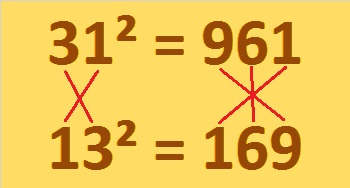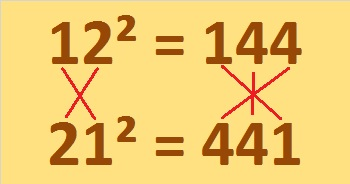# 961 is a Perfect Square in More Ways Than One

961 is a perfect square. Its square root is 31. Look what happens when their digits are reversed. We still get a perfect square!The digits 1, 6, and 9 form six different 3-digit numbers when each of those digits is used only one time. Here are some square facts about each of those numbers:

169 = 13²
196 = 14²
619 = 310² – 309²
691 = 346² – 345²
916 = 30² + 4²
961 = 31²

Of course, 169 also equals 5² + 12²

So 961 is a perfect square forward, backward, and upside-down!

My friend, Muthu Yuvaraj, shared a couple of similar square facts with me:andFascinating!

It is the sum of three consecutive square numbers:
313 + 317 + 331 = 961

It is the sum of five consecutive square numbers:
181 + 191 + 193 + 197 + 199 = 961

And it is the sum of the twenty-three prime numbers from 3 to 89.

It is palindrome 12321 in BASE 5 because 1(5⁴) + 2(5³) + 3(5²) + 2(5¹) + 1(5⁰) = 961

961 is a perfect square in base 10, and it looks like a perfect square in some other bases, too:
100 in BASE 31 because 1(31²) = 961
121 in BASE 30 because 1(30²) + 2(30¹) + 1(30⁰) = 961
144 in BASE 29 because 1(29²) + 4(29¹) + 4(29⁰) = 961
169 in BASE 28 because 1(28²) + 6(28¹) + 9(28⁰) = 961
441 in BASE 15 because 4(15²) + 4(15¹) + 1(15⁰) = 961
Remarkably, we saw 961, 169, 144, and 441 already in graphics for this post! So here’s one more graphic:• 961 is a composite number.
• Prime factorization: 961 = 31²
• The exponent in the prime factorization is 2. Adding one we get (2 + 1) = 3. Therefore 961 has exactly 3 factors.
• Factors of 961: 1, 31, 961
• Factor pairs: 961 = 1 × 961 or 31 × 31
• 961 is a perfect square. √961 = 31